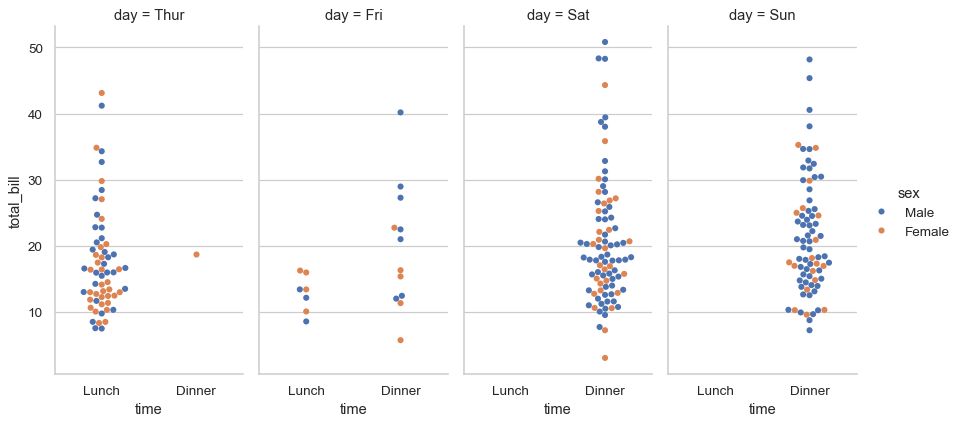# seaborn.swarmplot#

seaborn.swarmplot(data=None, *, x=None, y=None, hue=None, order=None, hue_order=None, dodge=False, orient=None, color=None, palette=None, size=5, edgecolor='gray', linewidth=0, hue_norm=None, native_scale=False, formatter=None, legend='auto', warn_thresh=0.05, ax=None, **kwargs)#

Draw a categorical scatterplot with points adjusted to be non-overlapping.

This function is similar to `stripplot()`, but the points are adjusted (only along the categorical axis) so that they don’t overlap. This gives a better representation of the distribution of values, but it does not scale well to large numbers of observations. This style of plot is sometimes called a “beeswarm”.

A swarm plot can be drawn on its own, but it is also a good complement to a box or violin plot in cases where you want to show all observations along with some representation of the underlying distribution.

Note

By default, this function treats one of the variables as categorical and draws data at ordinal positions (0, 1, … n) on the relevant axis. This can be disabled with the `native_scale` parameter.

Parameters:
dataDataFrame, array, or list of arrays, optional

Dataset for plotting. If `x` and `y` are absent, this is interpreted as wide-form. Otherwise it is expected to be long-form.

x, y, huenames of variables in `data` or vector data, optional

Inputs for plotting long-form data. See examples for interpretation.

order, hue_orderlists of strings, optional

Order to plot the categorical levels in; otherwise the levels are inferred from the data objects.

dodgebool, optional

When using `hue` nesting, setting this to `True` will separate the strips for different hue levels along the categorical axis. Otherwise, the points for each level will be plotted in one swarm.

orient“v” | “h”, optional

Orientation of the plot (vertical or horizontal). This is usually inferred based on the type of the input variables, but it can be used to resolve ambiguity when both `x` and `y` are numeric or when plotting wide-form data.

colormatplotlib color, optional

Single color for the elements in the plot.

palettepalette name, list, or dict

Colors to use for the different levels of the `hue` variable. Should be something that can be interpreted by `color_palette()`, or a dictionary mapping hue levels to matplotlib colors.

sizefloat, optional

Radius of the markers, in points.

edgecolormatplotlib color, “gray” is special-cased, optional

Color of the lines around each point. If you pass `"gray"`, the brightness is determined by the color palette used for the body of the points.

linewidthfloat, optional

Width of the gray lines that frame the plot elements.

native_scalebool, optional

When True, numeric or datetime values on the categorical axis will maintain their original scaling rather than being converted to fixed indices.

formattercallable, optional

Function for converting categorical data into strings. Affects both grouping and tick labels.

legend“auto”, “brief”, “full”, or False

How to draw the legend. If “brief”, numeric `hue` and `size` variables will be represented with a sample of evenly spaced values. If “full”, every group will get an entry in the legend. If “auto”, choose between brief or full representation based on number of levels. If `False`, no legend data is added and no legend is drawn.

axmatplotlib Axes, optional

Axes object to draw the plot onto, otherwise uses the current Axes.

kwargskey, value mappings

Other keyword arguments are passed through to `matplotlib.axes.Axes.scatter()`.

Returns:
axmatplotlib Axes

Returns the Axes object with the plot drawn onto it.

`boxplot`

A traditional box-and-whisker plot with a similar API.

`violinplot`

A combination of boxplot and kernel density estimation.

`stripplot`

A scatterplot where one variable is categorical. Can be used in conjunction with other plots to show each observation.

`catplot`

Combine a categorical plot with a `FacetGrid`.

Examples

Assigning a single numeric variable shows its univariate distribution with points adjusted along on the other axis such that they don’t overlap:

```tips = sns.load_dataset("tips")
sns.swarmplot(data=tips, x="total_bill")
```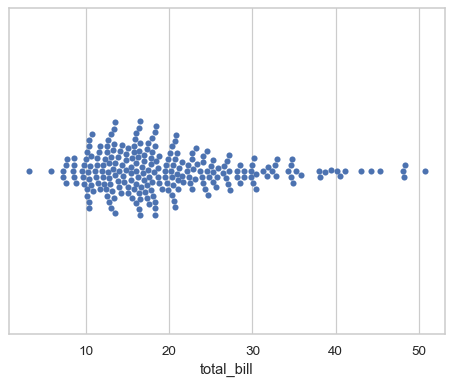Assigning a second variable splits the groups of points to compare categorical levels of that variable:

```sns.swarmplot(data=tips, x="total_bill", y="day")
```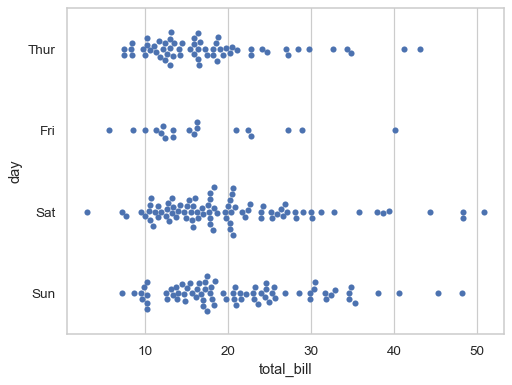Show vertically-oriented swarms by swapping the assignment of the categorical and numerical variables:

```sns.swarmplot(data=tips, x="day", y="total_bill")
```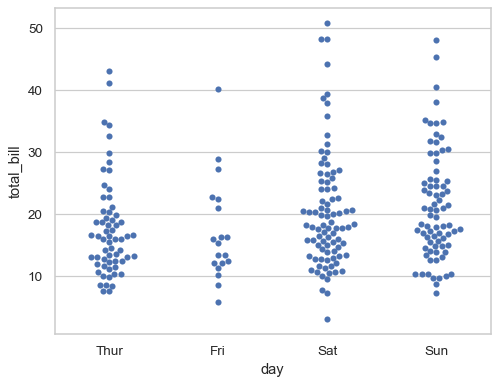Prior to version 0.12, the levels of the categorical variable had different colors by default. To get the same effect, assign the `hue` variable explicitly:

```sns.swarmplot(data=tips, x="total_bill", y="day", hue="day", legend=False)
```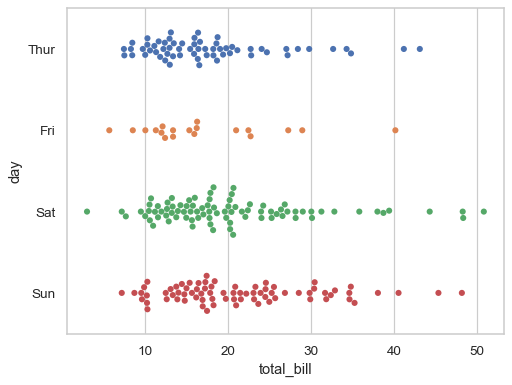Or you can assign a distinct variable to `hue` to show a multidimensional relationship:

```sns.swarmplot(data=tips, x="total_bill", y="day", hue="sex")
```If the `hue` variable is numeric, it will be mapped with a quantitative palette by default (note that this was not the case prior to version 0.12):

```sns.swarmplot(data=tips, x="total_bill", y="day", hue="size")
```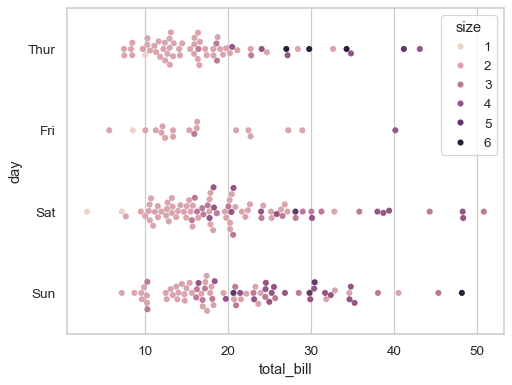Use `palette` to control the color mapping, including forcing a categorical mapping by passing the name of a qualitative palette:

```sns.swarmplot(data=tips, x="total_bill", y="day", hue="size", palette="deep")
```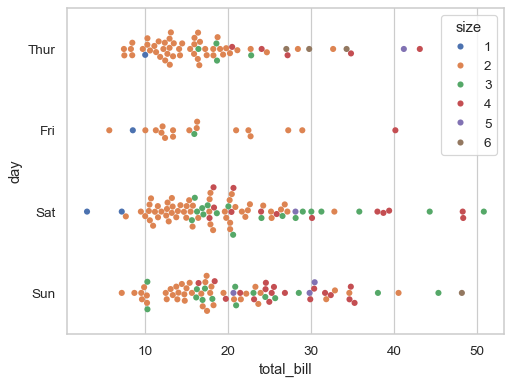By default, the different levels of the `hue` variable are intermingled in each swarm, but setting `dodge=True` will split them:

```sns.swarmplot(data=tips, x="total_bill", y="day", hue="sex", dodge=True)
```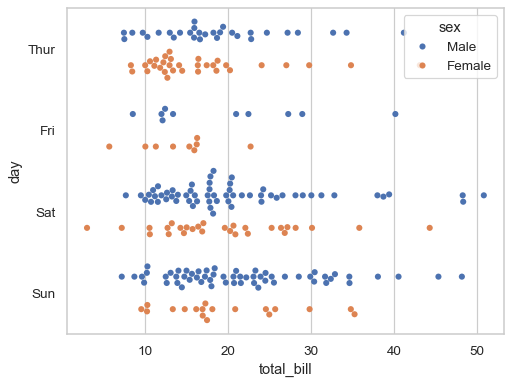The “orientation” of the plot (defined as the direction along which quantitative relationships are preserved) is usually inferred automatically. But in ambiguous cases, such as when both axis variables are numeric, it can be specified:

```sns.swarmplot(data=tips, x="total_bill", y="size", orient="h")
```
```/Users/mwaskom/code/seaborn/seaborn/categorical.py:3544: UserWarning: 15.4% of the points cannot be placed; you may want to decrease the size of the markers or use stripplot.
warnings.warn(msg, UserWarning)
/Users/mwaskom/code/seaborn/seaborn/categorical.py:3544: UserWarning: 17.3% of the points cannot be placed; you may want to decrease the size of the markers or use stripplot.
warnings.warn(msg, UserWarning)
```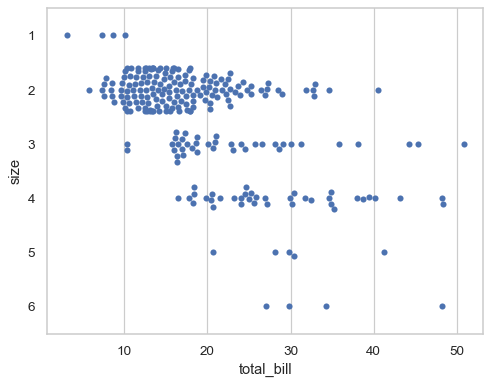When the local density of points is too high, they will be forced to overlap in the “gutters” of each swarm and a warning will be issued. Decreasing the size of the points can help to avoid this problem:

```sns.swarmplot(data=tips, x="total_bill", y="size", orient="h", size=3)
```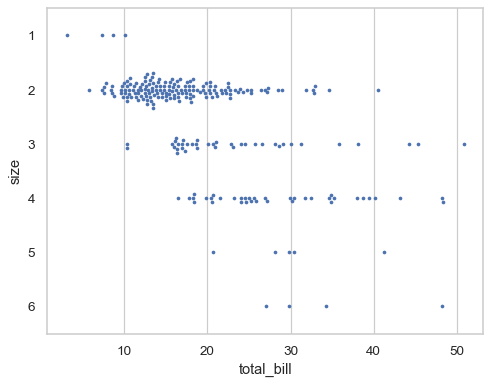By default, the categorical variable will be mapped to discrete indices with a fixed scale (0, 1, …), even when it is numeric:

```sns.swarmplot(
data=tips.query("size in [2, 3, 5]"),
x="total_bill", y="size", orient="h",
)
```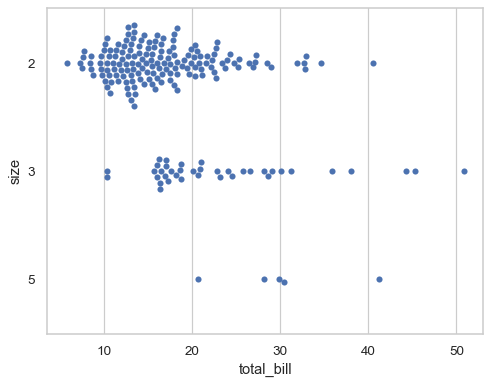To disable this behavior and use the original scale of the variable, set `native_scale=True` (notice how this also changes the order of the variables on the y axis):

```sns.swarmplot(
data=tips.query("size in [2, 3, 5]"),
x="total_bill", y="size", orient="h",
native_scale=True,
)
```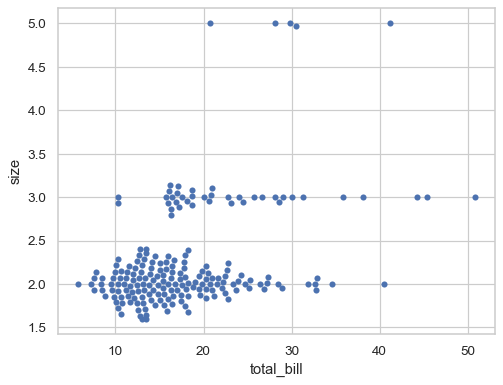Further visual customization can be achieved by passing keyword arguments for `matplotlib.axes.Axes.scatter()`:

```sns.swarmplot(
data=tips, x="total_bill", y="day",
marker="x", linewidth=1,
)
```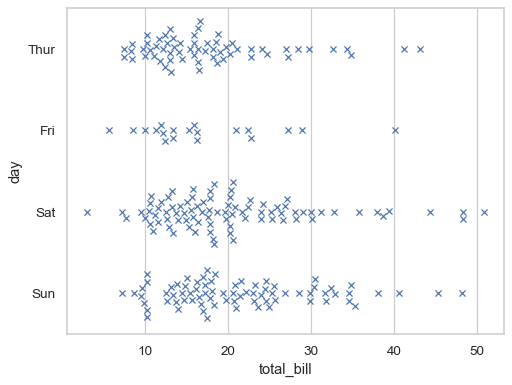To make a plot with multiple facets, it is safer to use `catplot()` with `kind="swarm"` than to work with `FacetGrid` directly, because `catplot()` will ensure that the categorical and hue variables are properly synchronized in each facet:

```sns.catplot(
data=tips, kind="swarm",
x="time", y="total_bill", hue="sex", col="day",
aspect=.5
)
```